# Geometric Shapes

In Mathematics, Geometric shapes are the figures which demonstrate the shape of the objects we see in our everyday life. In geometry, shapes are the forms of objects which have boundary lines, angles and surfaces. There are different types of 2d shapes and 3d shapes

Shapes are also classified with respect to their regularity or uniformity. A regular shape is usually symmetrical such as a square, circle, etc. Irregular shapes are asymmetrical. They are also called freeform shapes or organic shapes. For example, the shape of a tree is irregular or organic.

In plane geometry, the two-dimensional shapes are flat shapes and closed figures such as circles, squares, rectangles, rhombus, etc.  In solid geometry, the three-dimensional shapes are cube, cuboid, cone, sphere and cylinder. We can observe all these shapes in our daily existence also. For example books (cuboid shape), glasses (cylindrical shape), traffic cones (conical shape) and so on. In this article, you will learn different geometric shapes and their definition along with examples.

 To be Noted: A point has no dimension and a line is a one-dimensional shape. Both of these are the base of geometry. When two lines meet at a point, they form an angle where the point is said to be the vertex and lines are the arms.  The two-dimensional and three-dimensional shapes are formed using point, lines and angles.

Shapes are nothing but simple geometric figures which have a specific boundary, and interior and exterior surface area. In geometry, we can learn various shapes and their properties. Students are introduced with geometry in their classes with basic shapes and terms.

## Definition

Geometrical shapes are the figures which represent the forms of different objects. Some figures are two-dimensional, whereas some are three-dimensional shapes. The two-dimensional figures lie on only the x-axis and y-axis, but 3d shapes lie on the x, y and z axes. The z-axis shows the height of the object. As we have already discussed in the introduction, there are different shapes defined in geometry.

To draw or design any of these figures start with a line or a line segment or a curve. Depending upon the number and arrangement of these lines, we get different types of shapes and figures like a triangle, a figure where three line segments are connected, a pentagon (five-line segments) and so on. But every figure is not a complete figure.

## List of Geometric Shapes

Here is the list of different geometric shapes that we learn in geometry.

 Two Dimensional Shapes Three Dimensional Shapes Triangle Circle Semi-Circle Square Rectangle Parallelogram Rhombus Trapezium Kite Polygons (Pentagon, Hexagon, Octagon, Nonagon, Decagon, etc.) Sphere Cube Cuboid Cone Cylinder

## Types and Properties of Geometric Shapes

Go through the different types of shapes in geometry along with definitions here.

### Triangle

Triangle is a polygon, which is made of three sides and consists of three edges and three vertices. Also, the sum of its internal angles equals to 180o.

### Circle

Locus of all points at a fixed distance from a reference central point is called a Circle.

### Square

Square is a quadrilateral where all the four sides and angles are equal and the angles at all the vertices are equal to 90° each.

### Rectangle

A quadrilateral has two pairs of opposite sides equal in length and interior angles are at the right angles.

### Parallelogram

A parallelogram is a quadrilateral with two pairs of parallel sides and opposite angles are equal in measure.

### Polygons

These are made up of line segments and no curves. They are enclosed structures based on different lengths of sides and different angles.

### Figures of 2d Shapes

 Names of 2D Geometric Shapes Figure Circle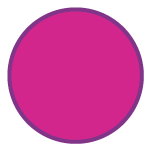Semicircle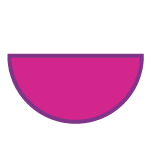Oval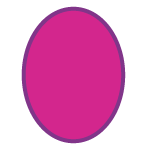Triangle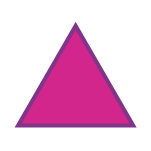Square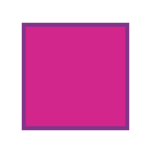RectangleParallelogram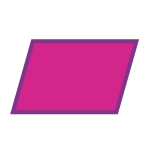Rhombus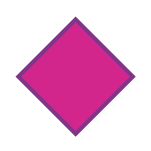Trapezium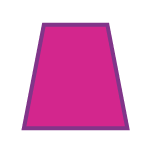Kite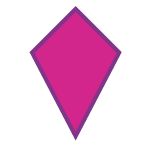Pentagon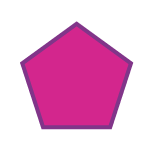Hexagon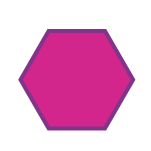Heptagon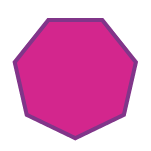Octagon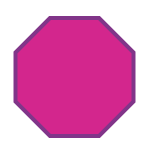Nonagon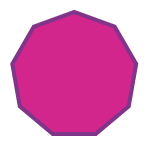Decagon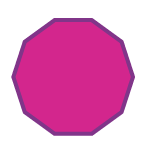### Three-Dimensional Shapes

Most of the three-dimensional shapes can be defined as a set of vertices, lines that connect the vertices and faces enclosed by these lines including obtained interior points. For many three dimensional shapes, faces are two-dimensional. Also, some shapes in three dimensions have curves surfaces. In three dimensions, the primary shapes are:

• Cube
• Cuboid
• Cone
• Cylinder
• Sphere

These can be better understood with the help of the table given below:

 Names of  3D geometric shapes Figure Definition Cube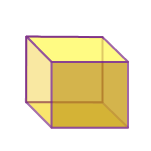A cube is a three-dimensional shape which has 6 faces, 8 vertices and 12 edges. The faces of the cube are square. Example: A Rubik’s cube Cuboid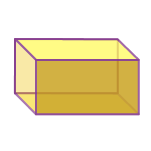A cuboid is also three dimensional solid having 6 faces, 8 vertices and 12 edges but the faces of the cuboid are in a rectangular shape.  Example: Matchbox Cone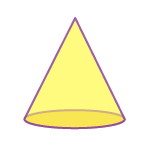A cone is a solid which has a circular base and narrows smoothly from the surface to the top at a point called apex or vertex.  Example: An icecream cone Cylinder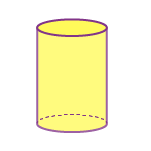A cylinder is a 3d solid shape that has two parallel circular bases connected by a curved surface. It has no vertex.  Example: Gas cylinder Sphere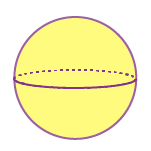A sphere is a round shape in a 3d plane, whose radius is extended to three dimensions (x-axis, y-axis and z-axis). Example: Ball

## Open and Closed Figures

A point is a small dot which is the starting point of a line segment. By definition, a line segment is a part of a line in which a narrow lane is connecting two points within a line. Different numbers of line segments give us different figures and such figures may be either open figures or closed shapes or figures.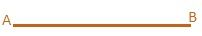### Closed Shapes

Geometric shapes such as a square, rectangles, and triangles are a few basic 2D shapes. These figures are collectively called polygons. A polygon is any flat shape or plane on a surface of a paper. They have a finite closed boundary made up of a fixed number of line segments and are called sides of the polygon. Each side meets at a common point called corners (vertex).

Such bounded geometric shapes like polygons are called closed figures. A boundary of a closed figure is not only made of line segments but also of curves. Hence, a closed figure can be defined as any geometric shape which starts and ends at the same point to form a boundary by line segments or by curves.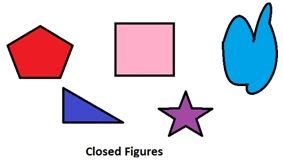### Open Shapes

Open figures are incomplete shapes. To sketch a closed figure one has to meet both the starting point and ending point. Open figures are also depicted by using line segments or by curves but at least the lines will be discontinuous. An open figure’s starting and endpoints are different.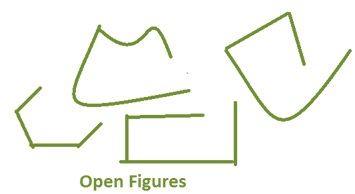### Different Shapes

In our daily existence, we may observe different shapes which look exactly the same as some three-dimensional geometric shapes.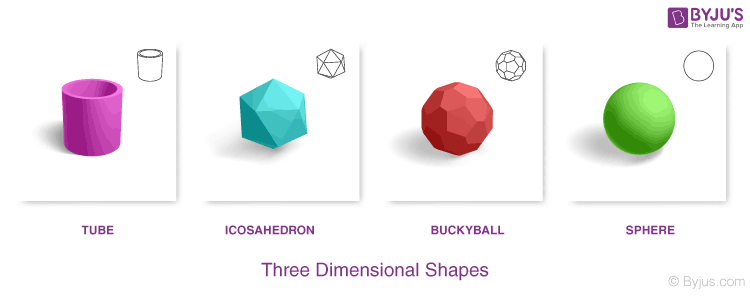Apart from the above examples, there are other objects in our surroundings such as traffic cones, Rubik’s cubes, pyramids and so on. Observe the below figure, to understand the different shapes that relate to geometric shapes.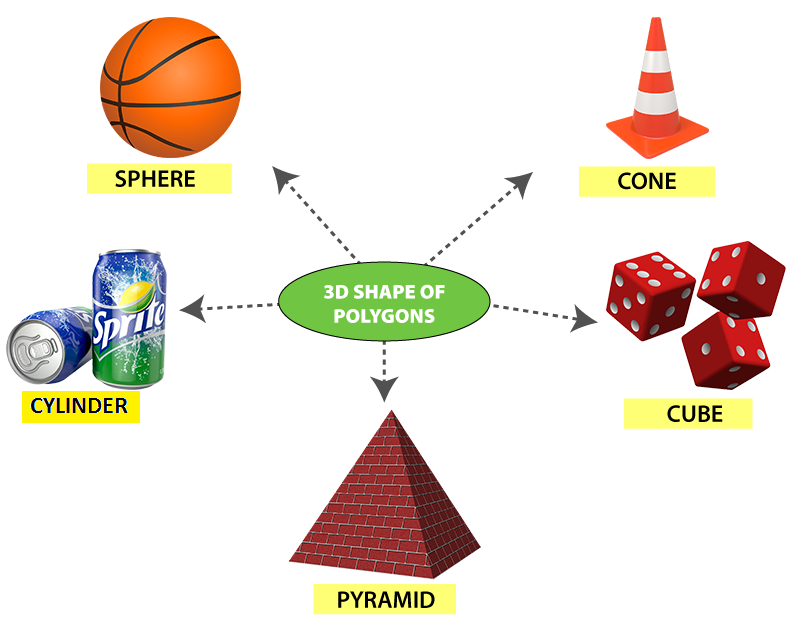### Solved Examples

Q.1: Find the open figure from the following.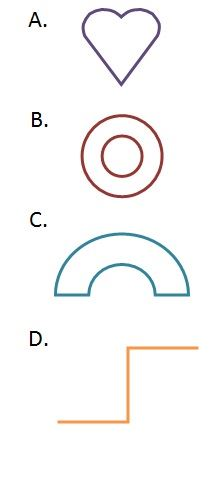Solution: Correct answer is figure D. All other figures are closed figures where both the start and endpoints are the same.

Q.2: How many sides does a decagon have?

1. 8
2. 9
3. 10
4. 11

A decagon has 10 sides and 10 vertices.

Q.3. Is a cone a two-dimensional shape or a three-dimensional shape?

Answer: A cone is a three-dimensional solid shape with a circular base and a single vertex. The surface of the cone is narrowed from the base to the vertex.

### Practice Problems

1. Name the geometrical shape that has five sides.
2. How many corners does a circle have?
3. What is the difference between a square, rectangle, and parallelogram?

Download BYJU’S- The Learning App and watch interactive videos. Also, take free tests to practise for exams.

## Frequently Asked Questions – FAQs

### What are the different geometric shapes in Maths?

There are many shapes in geometry based on their dimensions.
Circle, Triangle, Square, Rectangle, Kite, Trapezium, Parallelogram, Rhombus and different types of polygons are the 2-d shapes.
Cube, Cuboid, Sphere, Cone and Cylinder are the basic three-dimensional shapes.

### What are the types of triangles in geometry?

There are basically six types of triangles:
Scalene Triangle
Isosceles Triangle
Equilateral Triangle
Acute-angled triangle
Obtuse-angled triangle
Right-angled triangle

### What are the basic kinds of polygons?

The basic polygons are:
Triangle
Quadrilaterals – square, rectangle, parallelogram, trapezium, kite
Pentagon
Hexagon
Heptagon
Octagon
Nonagon
Decagon

### What are the examples of three-dimensional shapes?

Cube – Sugar cube, Rubik’s cube
Cuboid- A wooden rectangular box, matchbox
Cone- Icecream cone, Pyramid
Cylinder- Gas cylinder, Cylindrical jar

### What are the basic solid shapes?

The basic solid shapes are Cube, Cuboid, Cone, Sphere, Hemisphere and Cylinder.

Test your knowledge on Geometric Shapes